# The PLS Procedure

### Centering and Scaling

By default, the predictors and the responses are centered and scaled to have mean 0 and standard deviation 1. Centering the predictors and the responses ensures that the criterion for choosing successive factors is based on how much variation they explain, in either the predictors or the responses or both. (See the section Regression Methods for more details on how different methods explain variation.) Without centering, both the mean variable value and the variation around that mean are involved in selecting factors. Scaling serves to place all predictors and responses on an equal footing relative to their variation in the data. For example, if Time and Temp are two of the predictors, then scaling says that a change of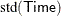in Time is roughly equivalent to a change of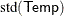in Temp.

Usually, both the predictors and responses should be centered and scaled. However, if their values already represent variation around a nominal or target value, then you can use the NOCENTER option in the PROC PLS statement to suppress centering. Likewise, if the predictors or responses are already all on comparable scales, then you can use the NOSCALE option to suppress scaling.

Note that, if the predictors involve crossproduct terms, then, by default, the variables are not standardized before standardizing the crossproduct. That is, if the ith values of two predictors are denoted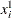and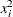, then the default standardized ith value of the crossproduct is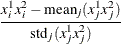If you want the crossproduct to be based instead on standardized variables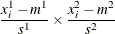where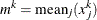and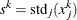for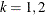, then you should use the VARSCALE option in the PROC PLS statement. Standardizing the variables separately is usually a good idea, but unless the model also contains all crossproducts nested within each term, the resulting model might not be equivalent to a simple linear model in the same terms. To see this, note that a model involving the crossproduct of two standardized variables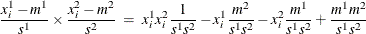involves both the crossproduct term and the linear terms for the unstandardized variables.

When cross validation is performed for the number of effects, there is some disagreement among practitioners as to whether each cross validation training set should be retransformed. By default, PROC PLS does so, but you can suppress this behavior by specifying the NOCVSTDIZE option in the PROC PLS statement.